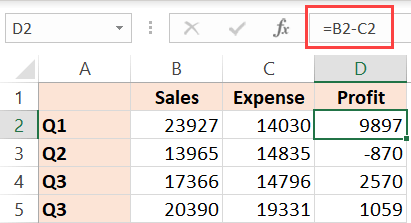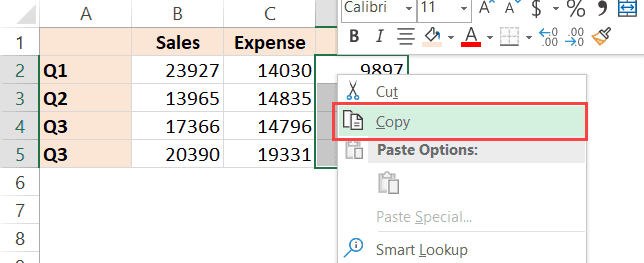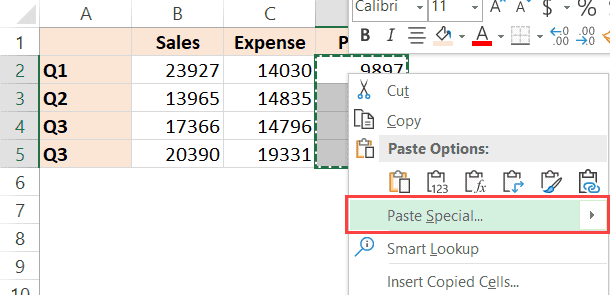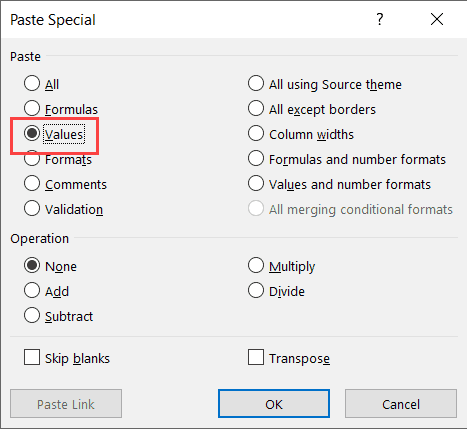# How to Remove Formulas in Excel (and keep the data)

Watch Video – How to Remove Formulas in Excel

Sometimes you may want to remove formulas from the worksheets but keep the data.

It is also recommended to remove formulas in case you don’t need these. This may also help you speed up your workbook.

In this short Excel tutorial, I will show you how to remove the formulas in Excel, but keep the data.

## Remove Formulas in Excel (and Keep the Data)

Suppose you have a dataset as shown below (where you have formulas in column B) and you want to remove the formulas but keep the data.Below are the steps to remove the formula while keeping the data:

1. Select the cells in column C that have the formula
2. Right-click on any of the selected cells and click on Copy (or use Control + C)3. Again click on any cell in the selection and right-click.
4. Click on the Paste Special option. This will open the paste special dialog box5. In the ‘Paste Special’ dialog box, select ‘Values’6. Click OK

The above steps would remove the formula from the selected cells and keep the values.

In case you prefer using the keyboard, you can also use the following shortcuts:

• Copy the cells – Control + C
• Paste as Values – ALT + E + S + V + Enter

In the above example, we have selected the range of cells from which we wanted to remove the formula. In case you want to remove it from all the cells in the worksheet, you can select all the cells and use the above steps)

In case you want to remove the formulas as well as the data, simply select the cells that have the formula and hit the Delete key,

Hope you found this Excel tutorial useful.

You may also like the following Excel tutorials: